Win up to 100% scholarship on Aakash BYJU'S JEE/NEET courses with ABNAT Win up to 100% scholarship on Aakash BYJU'S JEE/NEET courses with ABNAT

# Galvanometer

A galvanometer is a device that is used to detect a small electric current or measure its magnitude. The current and its intensity are usually indicated by a magnetic needle’s movement or that of a coil in a magnetic field, which is an important part of a galvanometer.

Since its discovery in the 1800s, the galvanometer has seen many iterations. Some of the different types of galvanometer include Tangent galvanometer, Astatic galvanometer, Mirror galvanometer and Ballistic galvanometer. However, today, the main type of galvanometer that is used widely is the D’Arsonval/Weston type or the moving coil type. A galvanometer is basically a historical name that has been given to a moving coil electric current detector.

## What Is a Moving Coil Galvanometer?

A moving coil galvanometer is an instrument which is used to measure electric currents. It is a sensitive electromagnetic device which can measure low currents even of the order of a few microamperes.

Moving-coil galvanometers are mainly divided into two types.

• Suspended coil galvanometer
• Pivoted-coil or Weston galvanometer

## Moving Coil Galvanometer Principle

A current-carrying coil, when placed in an external magnetic field, experiences magnetic torque. The angle through which the coil is deflected due to the effect of the magnetic torque is proportional to the magnitude of current in the coil.

## Moving Coil Galvanometer Construction and Diagram

The moving coil galvanometer is made up of a rectangular coil that has many turns, and it is usually made of thinly insulated or fine copper wire that is wounded on a metallic frame. The coil is free to rotate about a fixed axis. A phosphor-bronze strip that is connected to a movable torsion head is used to suspend the coil in a uniform radial magnetic field.

Essential properties of the material used for the suspension of the coil are conductivity and a low value of the torsional constant. A cylindrical soft iron core is symmetrically positioned inside the coil to improve the strength of the magnetic field and to make the field radial. The lower part of the coil is attached to a phosphor-bronze spring having a small number of turns. The other end of the spring is connected to binding screws.

The spring is used to produce a counter torque which balances the magnetic torque and hence helps in producing a steady angular deflection. A plane mirror which is attached to the suspension wire, along with a lamp and scale arrangement, is used to measure the deflection of the coil. The zero-point of the scale is at the centre.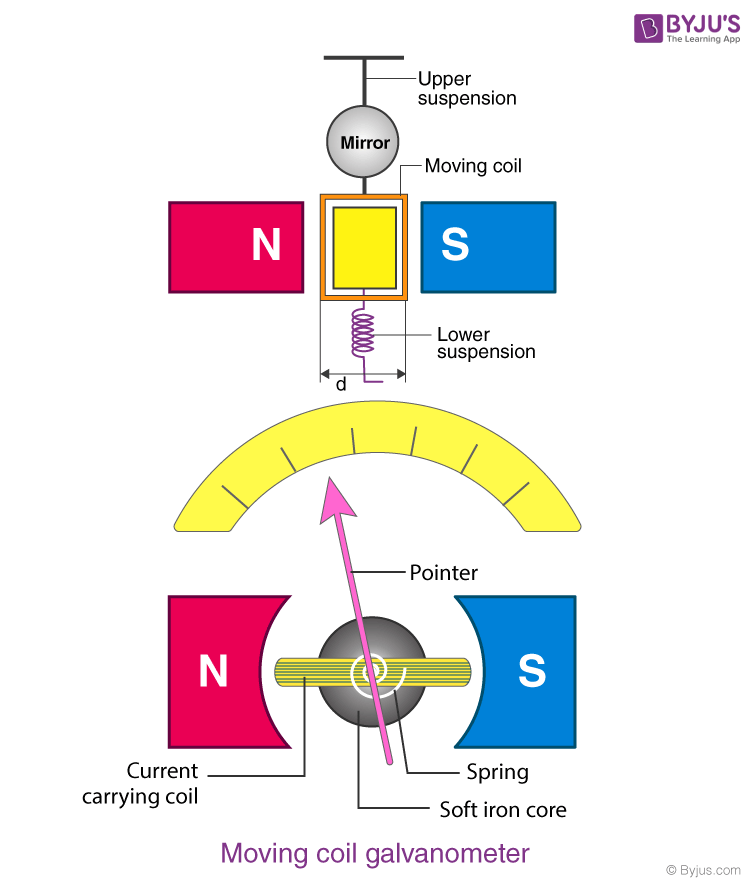## Working of Moving Coil Galvanometer

Let a current I flow through the rectangular coil of n number of turns and a cross-sectional area A. When this coil is placed in a uniform radial magnetic field B, the coil experiences a torque τ.

Let us first consider a single turn ABCD of the rectangular coil having a length l and breadth b. This is suspended in a magnetic field of strength B, such that the plane of the coil is parallel to the magnetic field. Since the sides AB and DC are parallel to the direction of the magnetic field, they do not experience any effective force due to the magnetic field. The sides AD and BC being perpendicular to the direction of the field, experience an effective force F given by F = BIl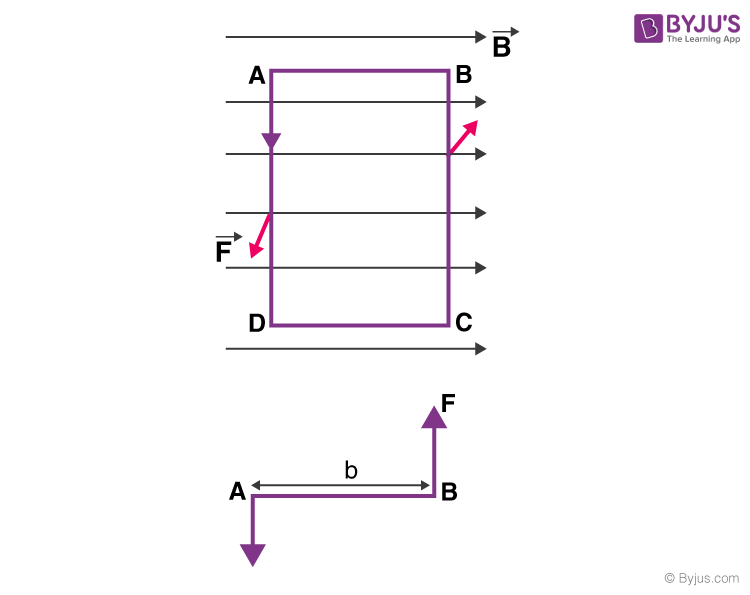Using Fleming’s left-hand rule, we can determine that the forces on AD and BC are in opposite directions to each other. When equal and opposite forces F called couple acts on the coil, it produces a torque. This torque causes the coil to deflect.

We know that torque τ = Force x Perpendicular distance between the forces

τ = F × b

Substituting the value of F, we already know,

Torque τ acting on single-loop ABCD of the coil = BIl × b

Where lx b is the area A of the coil,

Hence, the torque acting on n turns of the coil is given by

τ = nIAB

The magnetic torque thus produced causes the coil to rotate, and the phosphor bronze strip twists. In turn, the spring S attached to the coil produces a counter torque or restoring torque kθ, which results in a steady angular deflection.

Under an equilibrium condition,

kθ = nIAB

Here, k is called the torsional constant of the spring (restoring a couple per unit twist). The deflection or twist θ is measured as the value indicated on a scale by a pointer which is connected to the suspension wire.

θ= ( nAB / k)I

Therefore, θ ∝ I

The quantity nAB / k is a constant for a given galvanometer. Hence, it is understood that the deflection that occurs in the galvanometer is directly proportional to the current that flows through it.

Solved question: What is the purpose of introducing a cylindrical soft iron core inside the moving coil galvanometer?

Solution: The cylindrical soft iron core placed inside the galvanometer increases the magnetic field strength, thereby improving the sensitivity of the instrument. It also makes the magnetic field radial so that the angle between the plane of the coil and the magnetic lines of force remains zero at all times during the rotation of the coil.

## Sensitivity of Moving Coil Galvanometer

The general definition of the sensitivity experienced by a moving coil galvanometer is given as the ratio of change in deflection of the galvanometer to the change in current in the coil.

S = dθ/dI

The sensitivity of a galvanometer is higher if the instrument shows a larger deflection for a small value of current. Sensitivity is of two types, namely, current sensitivity and voltage sensitivity.

• Current Sensitivity

The deflection θ per unit current I is known as current sensitivity θ/I

θ/I = nAB/k

• Voltage Sensitivity

The deflection θ per unit voltage is known as voltage sensitivity θ/V. Dividing both sides by V in the equation θ= (nAB / k)I

θ/V= (nAB /V k)I = (nAB / k)(I/V) = (nAB /k)(1/R)

R stands for the effective resistance in the circuit.

It is worth noting that voltage sensitivity = Current sensitivity/Resistance of the coil. Therefore, under the condition that R remains constant, Voltage sensitivity ∝ Current sensitivity.

### Figure of Merit of a Galvanometer

It is the ratio of the full-scale deflection current and the number of graduations on the scale of the instrument. It is also the reciprocal of the current sensitivity of a galvanometer.

## Factors Affecting the Sensitivity of a Galvanometer

a) Number of turns in the coil

b) Area of the coil

c) Magnetic field strength B

d) The magnitude of a couple per unit twist k/nAB

Solved Question: Increase in current sensitivity results in an increase in voltage sensitivity of a moving coil galvanometer. Yes or no? Justify your answer.

Solution: No. An increase in the current sensitivity of a moving coil galvanometer may not necessarily result in an increase in voltage sensitivity. As the number of turns (length of the coil) is increased to increase the current sensitivity of the device, the resistance of the coil changes. This is because the resistance of the coil is dependent on factors like the length and area of the coil.

Also Read: Ohms Law and Resistance

As we know that voltage sensitivity θ/V = (nAB /k)(1/R); the overall value of voltage sensitivity remains unchanged.

## Applications of Galvanometer

The moving coil galvanometer is a highly sensitive instrument due to which it can be used to detect the presence of current in any given circuit. If a galvanometer is connected in a Wheatstone’s bridge circuit, the pointer in the galvanometer shows null deflection, i.e., no current flows through the device. The pointer deflects to the left or right depending on the direction of the current.

The galvanometer can be used to measure

a) The value of current in the circuit by connecting it in parallel to low resistance.

b) The voltage by connecting it in series with high resistance.

## Conversion of Galvanometer to Ammeter

A galvanometer is converted into an ammeter by connecting it in parallel with a low resistance called shunt resistance. Suitable shunt resistance is chosen depending on the range of the ammeter.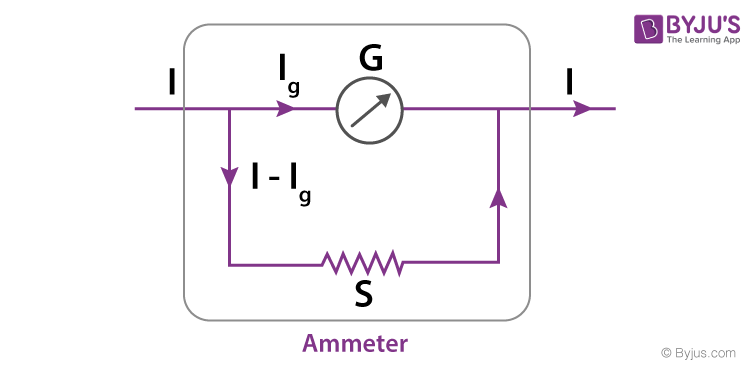In the given circuit,

RG – Resistance of the galvanometer

G – Galvanometer coil

I – Total current passing through the circuit

IG – Total current passing through the galvanometer, which corresponds to full-scale reading

Rs – Value of shunt resistance

When current IG passes through the galvanometer, the current through the shunt resistance is given by IS = I – IG. The voltages across the galvanometer and shunt resistance are equal due to the parallel nature of their connection.

Therefore, RG .IG= (I- IG).Rs

The value of S can be obtained using the above equation.

## Conversion of Galvanometer to Voltmeter

A galvanometer is converted into a voltmeter by connecting it in series with high resistance. A suitable high resistance is chosen depending on the range of the voltmeter.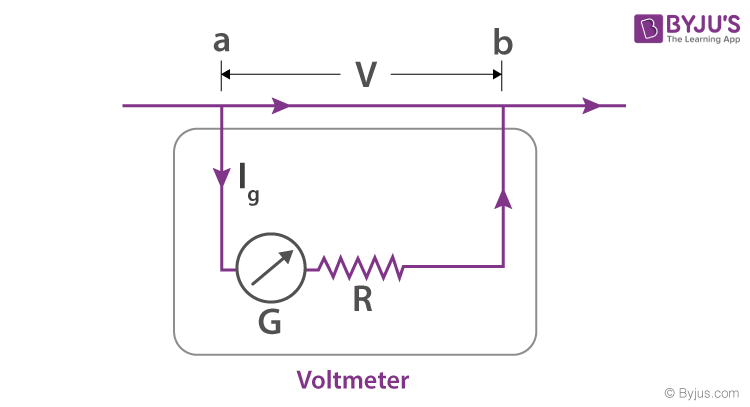In the given circuit,

RG = Resistance of the galvanometer

R = Value of high resistance

G = Galvanometer coil

I = Total current passing through the circuit

IG = Total current passing through the galvanometer, which corresponds to a full-scale deflection

V = Voltage drop across the series connection of galvanometer and high resistance

When current IG passes through the series combination of the galvanometer and the high resistance R, the voltage drop across the branch ab is given by

V= RG.IG + R.IG

The value of R can be obtained using the above equation.

Solved Question: A moving coil galvanometer of resistance 100Ω is used as an ammeter using a resistance of 0.1Ω. The maximum deflection current in the galvanometer is 100μA. Find the current in the circuit so that the ammeter shows maximum deflection. (IIT-JEE|2005)

Solution: It is given that RG =100Ω , Rs= 0.1Ω, IG =100μA

We know that RG .IG= (I- IG).RS

Therefore, I = (RG .IG+ IG.Rs)/ RS

I= (1+RG/ RS). IG

Substituting the given values, we get I= 100.1mA

Solved Question: A galvanometer coil of 40Ω resistance shows full range deflection for a current of 4mA. How can this galvanometer be converted into a voltmeter of range 0-12V?

Solution:As we know that V = IG (RG + R)

R = V/ IG – RG

= (12/ (4×10-3)) – 40

R = 2960 Ω

• High sensitivity
• Not easily affected by stray magnetic fields
• The torque to weight ratio is high
• High accuracy and reliability

• It can be used only to measure direct currents.
• Develops errors due to factors like ageing of the instrument, permanent magnets and damage of spring due to mechanical stress.

## Potentiometer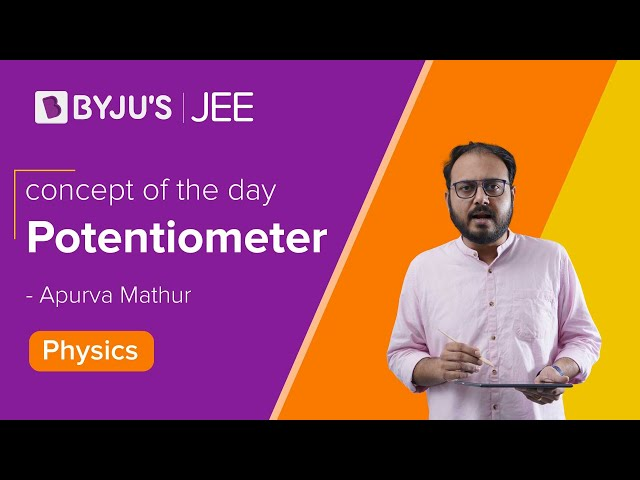## Frequently Asked Questions on Galvanometer

Q1

### What is the use of a galvanometer?

A galvanometer is used to measure the current and find the direction of the current. It can also measure voltage.

Q2

### Mention the working principle of a moving coil galvanometer.

The working principle of a moving coil galvanometer is when a current-carrying coil is placed in a magnetic field, it will experience a torque.

Q3

### Name the scientist who devised the galvanometer.

Johann Schweigger devised the earliest form of the electromagnetic galvanometer.

Q4

### How is a galvanometer connected to a circuit?

A galvanometer can be used as an ammeter or voltmeter, so it can be connected in series or parallel.